Win up to 100% scholarship on Aakash BYJU'S JEE/NEET courses with ABNAT Win up to 100% scholarship on Aakash BYJU'S JEE/NEET courses with ABNAT

# JEE Main 2022 July 26 – Shift 1 Chemistry Question Paper with Solutions

The JEE Main 2022 July 26 – Shift 1 Chemistry Question Paper with Solutions is given on this page. JEE Main 2022 question papers are solved by our experts at BYJU’S. Practising these question papers will be an added advantage for students’ exam preparation. JEE Main 2022 answer keys are very useful to determine their expected marks. Students can easily access and learn the JEE Main 2022 July 26 – Shift 1 Chemistry Question Paper with Solutions on this page. Revising the JEE Main 2022 question papers will help students to be stress-free during the exams.

## JEE Main 2022 26th July Shift 1 Chemistry Question Paper and Solutions

SECTION – A

Multiple Choice Questions: This section contains 20 multiple choice questions. Each question has 4 choices (1), (2), (3) and (4), out of which ONLY ONE is correct.

1. Match List-I with List-II.

List-I

(Compound)

List-II

(Shape)

(A) BrF5 (I) bent
(B) [CrF6]3– (II) square pyramidal
(C) O3 (III) trigonal bipyramidal
(D) PCI5 (IV) octahedral

Choose the correct answer from the options given below :

(A) (A)-(I), (B)-(II), (C)-(III), (D)-(IV)

(B) (A)-(IV), (B)-(III), (C)-(II), (D)-(I)

(C) (A)-(II), (B)-(IV), (C)-(I), (D)-(III)

(D) (A)-(III), (B)-(IV), (C)-(II), (D)-(I)

Sol. (A) BrF5 – square pyramidal

(B) [CrF6]3– – octahedral

(C) O3– bent

(D) PCI5– trigonal bipyramidal

2. Match List-I with List-II.

List-I

(Processes/ Reactions)

List-II

(Catalyst)

(A) 2SO2(g) + O2(g) (I) Fe(s) → 2SO3(g)
(B) 4NH3(g) + 5O2(g) (II) Pt(s) – Rh(s) → 4NO(g) + 6H2O(g)
(C) N2(g) + 3H2(g) (III) V2O5 → 2NH3(g)
(D) Vegetable oil (l) + H2 (IV) Ni(s) → Vegetable ghee(s)

Choose the correct answer from the options given below :

(A) (A)-(III), (B)-(I), (C)-(II), (D)-(IV)

(B) (A)-(III), (B)-(II), (C)-(I), (D)-(IV)

(C) (A)-(IV), (B)-(III), (C)-(I), (D)-(II)

(D) (A)-(IV), (B)-(II), (C)-(III), (D)-(I)

Sol.

$$\begin{array}{l}\left(A\right) 2SO_2(g) + O_2(g)\xrightarrow[~~~~~~~]{\text{v}_2\text{o}_5} 2SO_3\end{array}$$
$$\begin{array}{l}\left(B\right) 4NH_3(g) + 5O_2(g)\xrightarrow[~~~~~~~~~~~~~~~]{\text{Pt(s)-Rh(s)}} 4NO(g) + 6H_2O(g)\end{array}$$
$$\begin{array}{l}\left(C\right) N_2(g) + 3H_2(g) \xrightarrow[~~~~~~~~~~~~~~~]{\text{Fe(s)}} 2NH_3(g)\end{array}$$
$$\begin{array}{l}\left(D\right) \text{Vegetable oil(l)} + H_2\xrightarrow[~~~~~~~~~~~~~~~]{\text{Ni(s)}} \text{Vegetable ghee(s)}\end{array}$$

3. Given two statements below:

Statement I : In Cl2 molecule the covalent radius is double of the atomic radius of chlorine.

Statement II :Radius of anionic species is always greater than their parent atomic radius.

Choose the most appropriate answer from options given below:

(A) Both Statement I and Statement II are correct.

(B) Both Statement I and Statement II are incorrect.

(C) Statement I is correct but Statement II is incorrect.

(D) Statement I is incorrect but Statement II is correct.

Sol.

• Radius of anionic species is always greater than their parent atomic radius as nuclear charge decreases in anionic counterpart.

4. Refining using the liquation method is the most suitable for metals with:

(A) Low melting point

(B) High boiling point

(C) High electrical conductivity

(D) Less tendency to be soluble in melts than impurities

Sol. Refining using the liquation method is the most suitable for metals with a low melting point.

5. Which of the following can be used to prevent the decomposition of H2O2?

(A) Urea

(B) Formaldehyde

(C) Formic acid

(D) Ethanol

Sol. Urea is used as a stabilizer for the storage of H2O2.

6. Reaction of BeCl2 with LiAlH4gives :

(A) AlCl3

(B) BeH2

(C) LiH

(D) LiCl

(E) BeAlH4

Choose the correct answer from the options given below :

(A) (A), (D) and (E)

(B) (A), (B) and (D)

(C) (D) and (E)

(D) (B), (C) and (D)

Sol. 2BeCl2 + LiAlH4→ 2BeH2 + LiCl + AlCl3

7. Borazine, also known as inorganic benzene, can be prepared by the reaction of 3-equivalents of “X” with 6-equivalents of “Y”. “X” and “Y”, respectively are:

(A) B(OH)3 and NH3

(B) B2H6 and NH3

(C) B2H6 and HN3

(D) NH3 and B2O3

Sol.

$$\begin{array}{l} 3B_2H_6+6NH_3\rightarrow \underset{\text{(Borazine)}}{2B_3N_3H_6} \end{array}$$

8. Which of the given reactions is not an example of a disproportionation reaction?

$$\begin{array}{l}\left(A\right) 2H_2O_2\rightarrow 2H_2O + O_2\end{array}$$
$$\begin{array}{l}\left(B\right) 2NO_2 + H_2O \rightarrow HNO_3 + HNO_2\end{array}$$
$$\begin{array}{l} (\text{C})\ MnO_4^-+4H^++3e^-\rightarrow MnO_2+2H_2O\end{array}$$
$$\begin{array}{l} (\text{D})\ 3MnO_4^{2-}+4H^+\rightarrow 2MnO_4^-+MnO_2+2H_2O\end{array}$$

Sol.

$$\begin{array}{l} \overset{+7}{M}nO_4^-+4H^++3e^-\rightarrow \overset{+4}{M}nO_2+2H_2O\end{array}$$
$$\begin{array}{l} \text{The above reaction involves the reduction of}~MnO_4^- \text{to}~ MnO_2.\end{array}$$

9. The dark purple colour of KMnO4 disappears in the titration with oxalic acid in acidic medium. The overall change in the oxidation number of manganese in the reaction is :

(A) 5

(B) 1

(C) 7

(D) 2

Sol.

$$\begin{array}{l} 2K\overset{+7}{M}nO_4+5H_2C_2O_4+3H_2SO_4\rightarrow \end{array}$$
$$\begin{array}{l} K_2SO_4+2\overset{+2}{M}nSO_4+10CO_2+8H_2O\end{array}$$

Change is oxidation state Mn is 5.

10.

$$\begin{array}{l} \overset{\bullet}{C}l+CH_4\rightarrow A+B \end{array}$$

A and B in the above atmospheric reaction step are:

$$\begin{array}{l}\left (A\right) C_2H_6~\text{and}~ Cl_2\end{array}$$
$$\begin{array}{l} (\text{B})\ \overset{\bullet}{C}HCl_2\text{ and }H_2\end{array}$$
$$\begin{array}{l} (\text{C})\ \overset{\bullet}{C}H_3\text{ and }HCl\end{array}$$
$$\begin{array}{l}\left(D\right) C_2H_6~ \text{and}~ HCl\end{array}$$

Sol.

$$\begin{array}{l} \overset{\bullet}{C}l+CH_4\rightarrow \underset{(\text{A})}{\overset{\bullet}{C}H_3}+\underset{(\text{B})}{HCl}\end{array}$$

11. Which technique among the following, is most appropriate in separation of a mixture of100 mg of p-nitrophenol and picric acid?

(A) Steam distillation

(B) 2-5 ft long column of silica gel

(C) Sublimation

(D) Preparative TLC (Thin Layer Chromatography)

Sol. Thin layer chromatography is a technique used to isolate non-volatile mixtures.

Hence, mixture of p-nitrophenol and Picric acid is separated by TLC.

12. The difference in the reaction of phenol with bromine in chloroform and bromine in water medium is due to:

(A) Hyperconjugation in substrate

(B) Polarity of solvent

(D) Electromeric effect of substrate

Sol. Phenol gives different products with bromine in chloroform and water medium due to the polarity difference between chloroform and water acting as a solvent.

13. Which of the following compounds is not aromatic?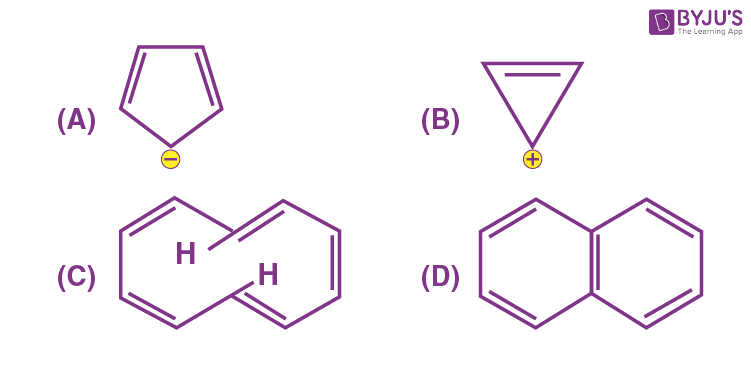Sol.is a non-planar compound; hence it is not aromatic.

14. The products formed in the following reaction, A and B are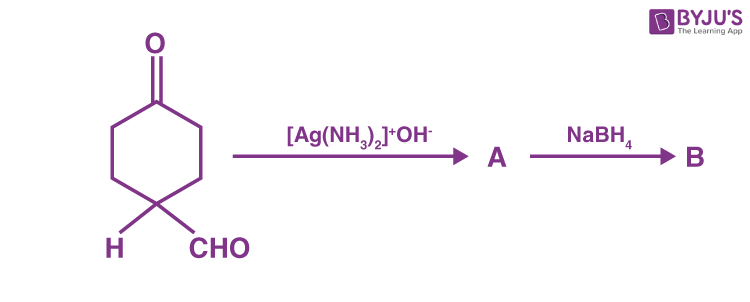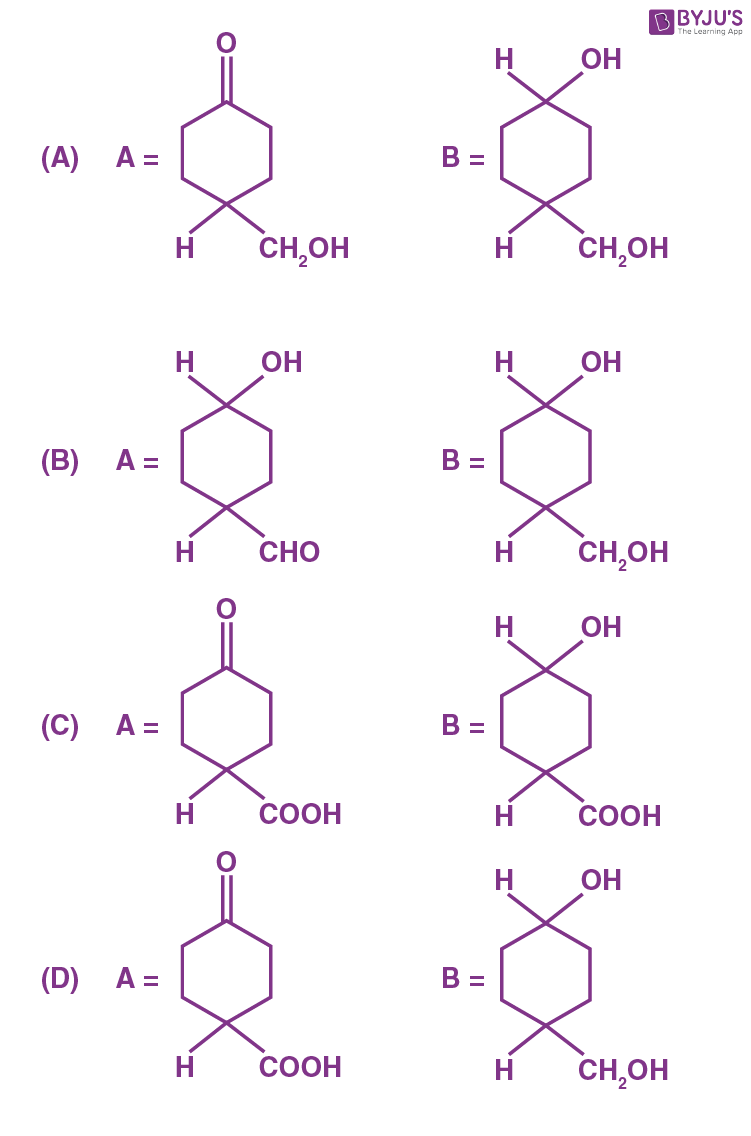Sol.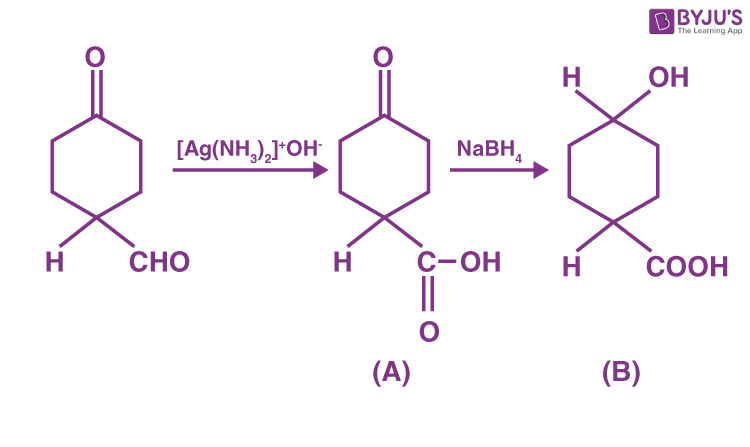15. Which reactant will give the following alcohol on reaction with one mole of phenyl magnesium bromide (PhMgBr) followed by acidic hydrolysis?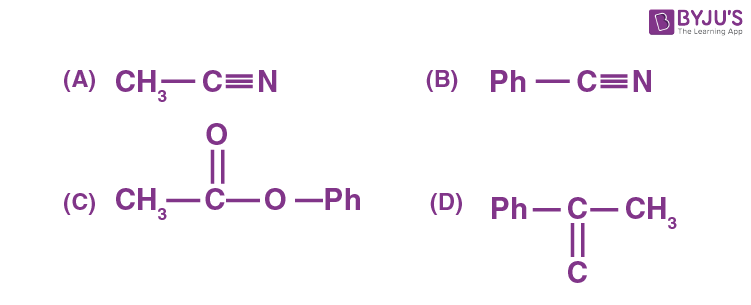Sol.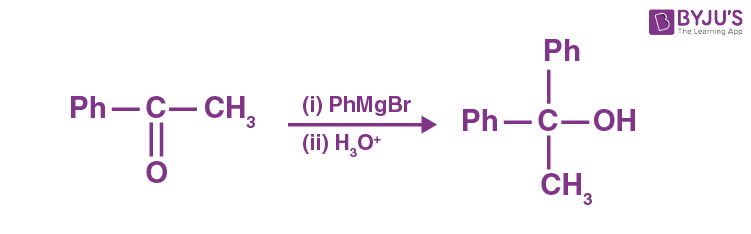16. The major product of the following reaction is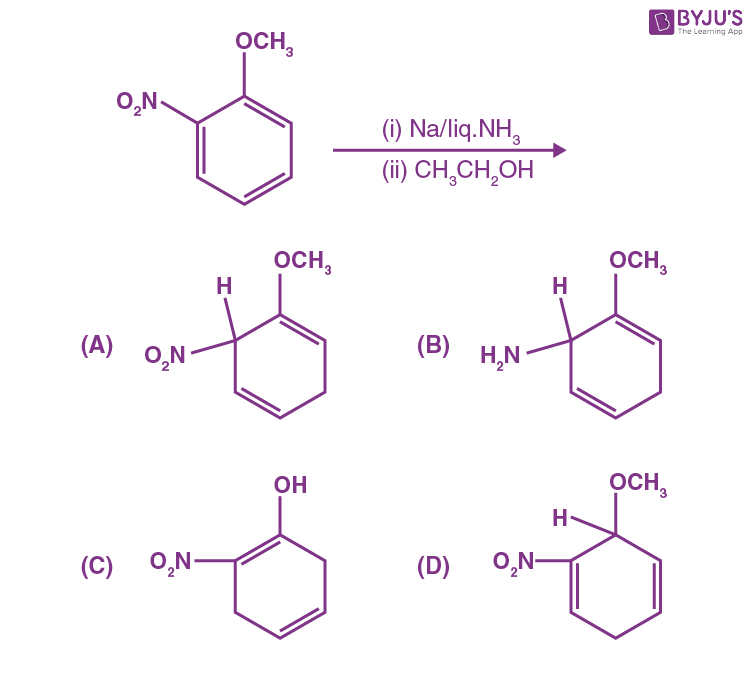Sol.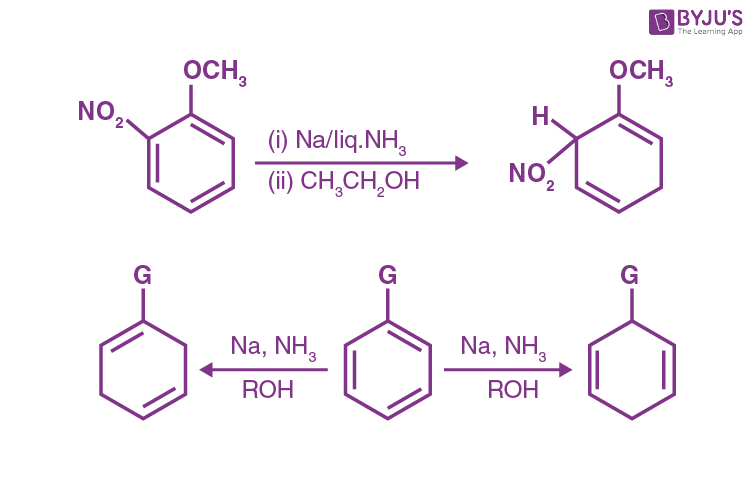(When G = EDG) (When G = EWG)

EDG → Electron donating group

EWG → Electron withdrawing group

17. The correct stability order of the following diazonium salt is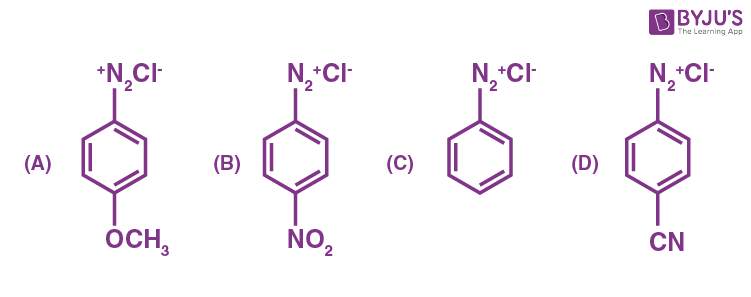(A) (A) > (B) > (C) > (D)

(B) (A) > (C) > (D) > (B)

(C) (C) > (A) > (D) > (B)

(D) (C) > (D) > (B) > (A)

Sol. Diazonium salt containing aryl group directly linked to electron donating group is the most stable due to resonance. The +M effect stabilizes the intermediate, whereas Electron withdrawing group on benzene destabilizes the intermediate at the para position.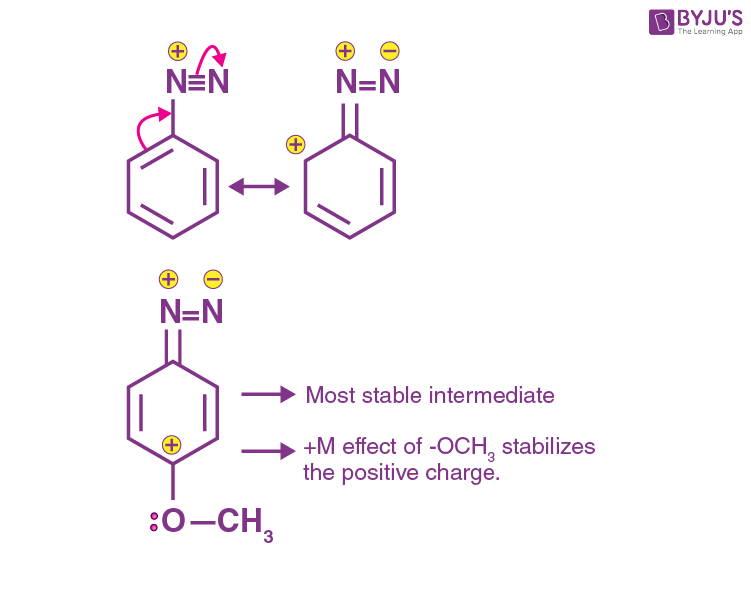Order will be A > C > D > B.

18. Stearic acid and polyethylene glycol react to form which one of the following soap/s detergents?

(A) Cationic detergent

(B) Soap

(C) Anionic detergent

(D) Non-ionic detergent

Sol.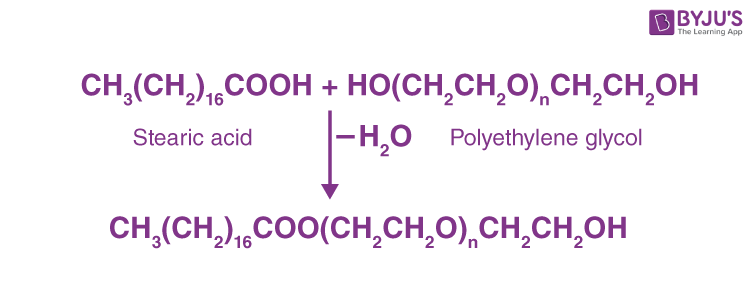The product do not contain any ion in its constitution hence it is a non-ionic detergent.

19. Which one of the following is a reducing sugar?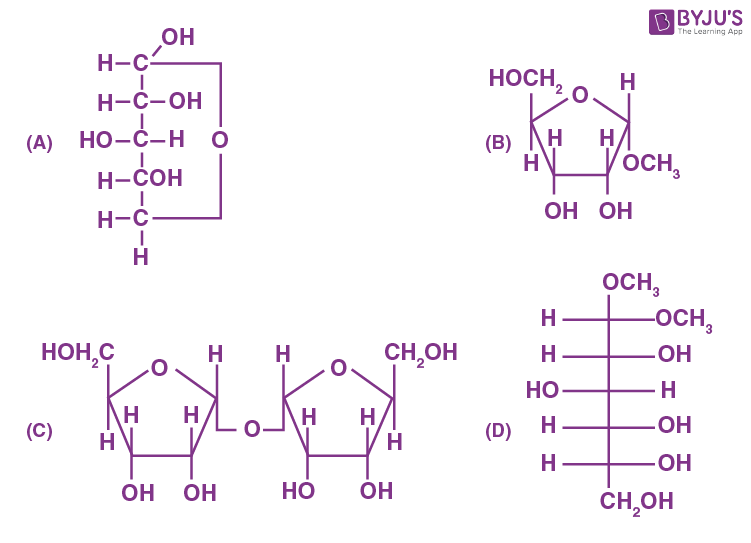Sol.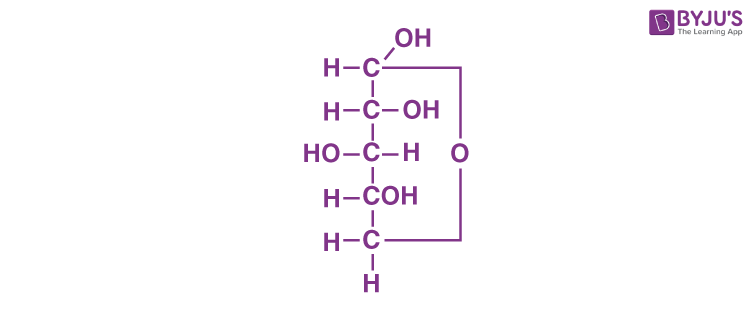The sugar gives +veTollen’s test hence it’s a reducing sugar.

20. Given below are two statements: one is labelled as Assertion (A) and the other is labelled as Reason (R).

Assertion (A): Experimental reaction of CH3Cl with aniline and anhydrous AlCl3 does not give
o and p-methylaniline.

Reason (R): The –NH2 group of aniline becomes deactivating because of salt formation with anhydrous AlCl3 and hence yields m-methyl aniline as the product.

In the light of the above statements, choose the most appropriate answer from the options given below :

(A) Both (A) and (R) are true and (R) is the correct explanation of (A).

(B) Both (A) and (R) are true but (R) is not the correct explanation of (A).

(C) (A) is true, but (R) is false.

(D) (A) is false, but (R) is true.

Sol.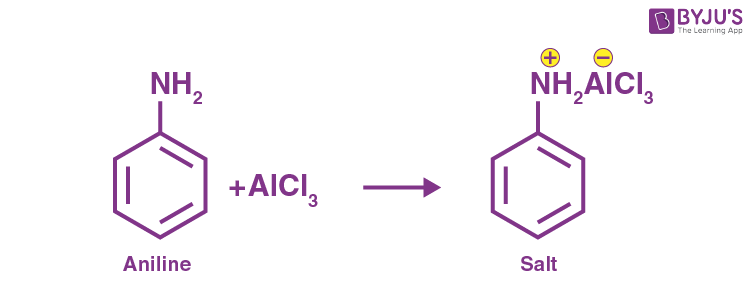Aniline does not undergo FriedelCraft reaction because the reagent AlCl3 being electron deficient acts as a Lewis acid.

SECTION – B

Numerical Value Type Questions: This section contains 10 questions. In Section B, attempt any five questions out of 10. The answer to each question is a NUMERICAL VALUE. For each question, enter the correct numerical value (in decimal notation, truncated/rounded-off to the second decimal place; e.g. 06.25, 07.00, –00.33, –00.30, 30.27, –27.30) using the mouse and the on-screen virtual numeric keypad in the place designated to enter the answer.

1. Chlorophyll extracted from the crushed green leaves was dissolved in water to make 2 L solution of Mg of concentration 48 ppm. The number of atoms of Mg in this solution is x × 1020 atoms. The value of x is ________. (Nearest integer)

(Given : Atomic mass of Mg is 24 g mol–1; NA = 6.02 × 1023 mol–1)

Sol. In 2L → 96 mg of Mg

$$\begin{array}{l}\text{Number of atoms of Mg} =\frac{96\times 10^{-3}}{24}\times N_A\end{array}$$
$$\begin{array}{l} =4\times 10^{-3}\times 6\times 10^{23} \\ = 24 \times 10^{20}\\x = 24\end{array}$$

2. A mixture of hydrogen and oxygen contains 40% hydrogen by mass when the pressure is 2.2 bar. The partial pressure of hydrogen is _______ bar. (Nearest integer)

Sol. 40% w/w hydrogen gas is given in mixture of H2 and oxygen.

Wt. of H2 = 40 g

Wt. of O2 = 60 g

$$\begin{array}{l} \chi_{H_2}=\frac{n_{H_2}}{n_{H_2}+n_{O_2}}\end{array}$$
$$\begin{array}{l} =\frac{\frac{40}{2}}{\frac{40}{2}+\frac{60}{32}}\end{array}$$
$$\begin{array}{l} =\frac{20}{20+1.875}\end{array}$$
$$\begin{array}{l} =\frac{20}{21.875}=0.914\end{array}$$
$$\begin{array}{l} P_{H_2}=\chi _{H_2}\times P_T \\ = 0.914 \times 2.2\end{array}$$
$$\begin{array}{l} =2.01\simeq 2\text{ bar}\end{array}$$

3. The wavelength of an electron and a neutron will become equal when the velocity of the electron is x times the velocity of neutron. The value of x is ______. (Nearest integer)

(Mass of electron is 9.1 × 10–31 kg and mass of neutron is 1.6 × 10–27 kg)

Sol.

$$\begin{array}{l} \lambda_e=\frac{h}{m_e\times V_e},\ \lambda_N=\frac{h}{m_N\times V_N}\end{array}$$
$$\begin{array}{l} \lambda_e=\lambda_N~~~~~~~\text{when }V_e=xV_N \end{array}$$
$$\begin{array}{l} \frac{1}{m_eV_e}=\frac{1}{m_N\times V_N}\end{array}$$
$$\begin{array}{l} \frac{m_N}{m_e}=\frac{V_e}{V_N}=x\end{array}$$
$$\begin{array}{l} x=\frac{1.6\times 10^{-27}}{9.1\times 10^{-31}}= 0.17582 \times 10^4\end{array}$$
$$\begin{array}{l} \simeq 1758\end{array}$$

4. 2.4 g coal is burnt in a bomb calorimeter in excess of oxygen at 298 K and 1 atm pressure. The temperature of the calorimeter rises from 298 K to 300 K. The enthalpy change during the combustion of coal is –x kJ mol–1. The value of x is ______. (Nearest integer)

(Given : Heat capacity of bomb calorimeter is 20.0 kJ K–1. Assume coal to be pure carbon)

Sol.

$$\begin{array}{l} Q\left(\text{Heat evolved}\right)=-\frac{C_{\text{system }}\Delta T}{n}\end{array}$$
$$\begin{array}{l} n_\text{coal}=\frac{2.4}{12}\end{array}$$
$$\begin{array}{l} Q=\frac{-20\left(300-298\right)}{0.2}\end{array}$$
$$\begin{array}{l} Q=-200\text{ kJ/mol} \\x = 200\end{array}$$

5. When 800 mL of 0.5 M nitric acid is heated in a beaker, its volume is reduced to half and 11.5 g of nitric acid is evaporated. The molarity of the remaining nitric acid solution is x × 10–2 M. (Nearest integer)

(Molar mass of nitric acid is 63 g mol–1)

Sol.

$$\begin{array}{l}\text{m moles of HNO}_3 = 800 \times 0.5\end{array}$$
$$\begin{array}{l}\text{Moles of HNO}_3 = 400 \times 10^{-3} = 0.4 ~\text{moles}\end{array}$$

Weight of HNO3 = 0.4 × 63 g

= 25.2 g

Remaining acid = 25.2 – 11.5

= 13.7 g

$$\begin{array}{l} M=\frac{13.7\times1000}{400\times63} \end{array}$$
$$\begin{array}{l} =\frac{137}{252}=0.54\\= 54 \times 10^{-2}\end{array}$$

6. At 298 K, the equilibrium constant is 2 × 1015 for the reaction:

$$\begin{array}{l}Cu(s) + 2Ag^+(aq)\rightleftharpoons Cu^{2+}(aq) + 2Ag(s)\end{array}$$

The equilibrium constant for the reaction

$$\begin{array}{l} \frac{1}{2}Cu^{2+}\left(aq\right)+Ag\left(s\right)\rightleftharpoons \frac{1}{2}Cu\left(s\right)+Ag^+\left(aq\right)\times{is}~ x \times 10^{-8}.\end{array}$$
The value of x is ________
(Nearest integer)

Sol.

$$\begin{array}{l}Cu(s) + 2Ag^+(aq) \rightleftharpoons Cu^{2+}(aq) + 2Ag(s)\end{array}$$

k = 2 × 1015

$$\begin{array}{l} \frac{1}{2}Cu\left(s\right)+Ag^+\left(aq\right)\rightleftharpoons Cu^{2+}\left(aq\right)+2Ag\left(s\right)\end{array}$$
$$\begin{array}{l} K’=\frac{1}{\left(K\right)^\frac{1}{2}}=\frac{1}{\left(2\times10^{15}\right)^\frac{1}{2}}= 2.23 \times 10^{–8}\end{array}$$
$$\begin{array}{l} x\simeq 2\end{array}$$

7. The amount of charge in F(Faraday) required to obtain one mole of iron from Fe3O4 is __________. (Nearest integer)

Sol.

$$\begin{array}{l}For Fe_3O_4,\\x=\frac{+8}{3} \end{array}$$

where x is oxidation state of Fe.

$$\begin{array}{l} Fe_3O_4+8H^++8e^-\rightarrow 3Fe+4H_2O\end{array}$$
$$\begin{array}{l}\text{Charge required =}\frac{8}{3}\times F=\frac{8F}{3}\simeq 3F\end{array}$$

8. For a reaction A → 2B + C the half lives are 100 s and 50 s when the concentration of reactant A is 0.5 and 1.0 mol L–1, respectively. The order of the reaction is ________. (Nearest integer)

Sol.

$$\begin{array}{l} t_\frac{1}{2}\propto \frac{1}{\left(a_0\right)^{n-1}} \end{array}$$
$$\begin{array}{l}t_{\frac{1}{2}}= 100\ \text{sec}\ a_0 = 0.5\end{array}$$
$$\begin{array}{l}t_{\frac{1}{2}}= 50\ \text{sec}\ a_0 = 1\end{array}$$
$$\begin{array}{l} \frac{100}{50}=\left(\frac{1}{0.5}\right)^{n-1}\end{array}$$
$$\begin{array}{l}(2) = (2)^{n-1}\end{array}$$

n–1 = 1

n = 2

9. The difference between spin only magnetic moment value of [Co(H2O)6]Cl2 and [Cr(H2O)6]Cl3is _________.

Sol. Co → 4s2 3d7

H2O is weak field ligand.

$$\begin{array}{l}Co^{2+}\rightarrow 3d^7\\n = 3~~~~\mu_1=\sqrt{n\left(n+2\right)} \end{array}$$
$$\begin{array}{l} =\sqrt{15}\text{ B.M.}\end{array}$$

Cr →4s1 3d5

$$\begin{array}{l}Co^{3+}\rightarrow 3d^3 ~~~~~~n = 3\\ \mu_2=\sqrt{15}\text{ B.M.}\\\mu_1 – \mu_2 = 0\end{array}$$

10. In the presence of sunlight, benzene reacts with CI2 to give product X. The number of hydrogens in X is _________.

Sol.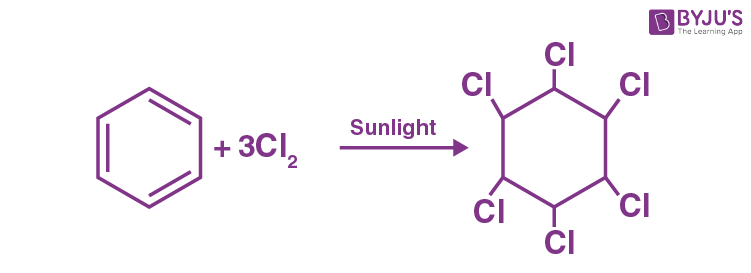Total number of hydrogens is six.

### Download PDF of JEE Main 2022 July 26 Shift 1 Chemistry Paper & Solutions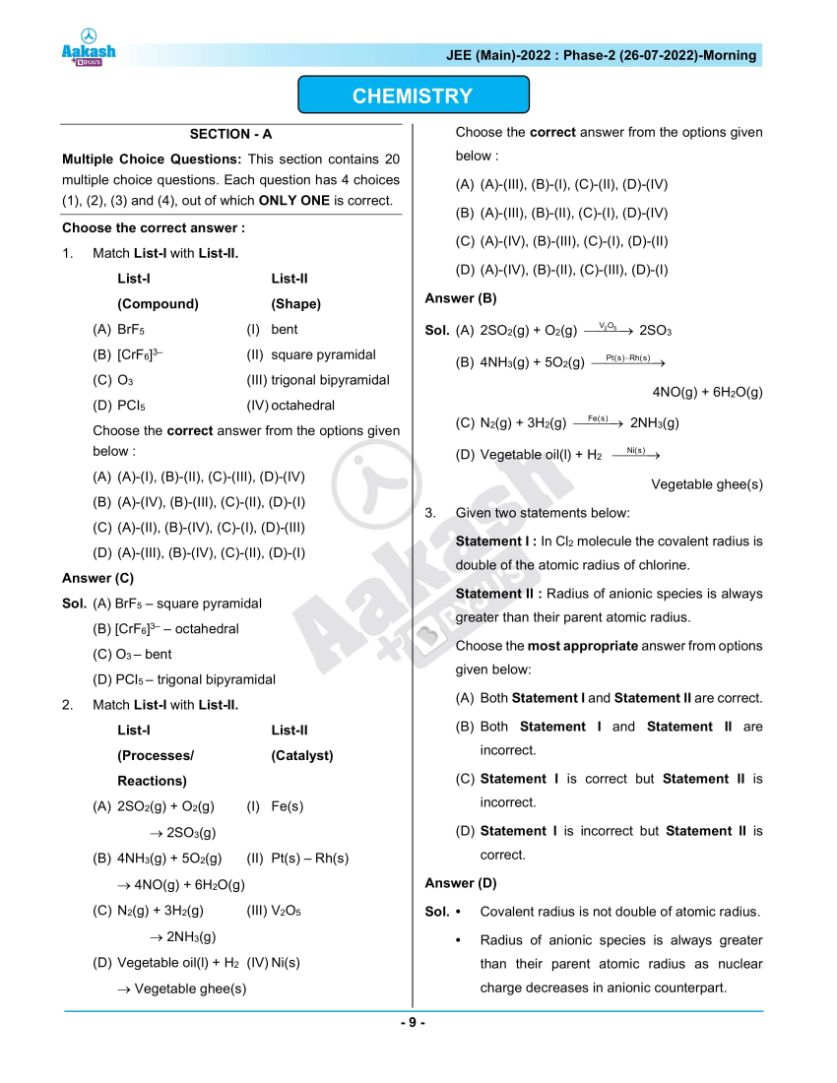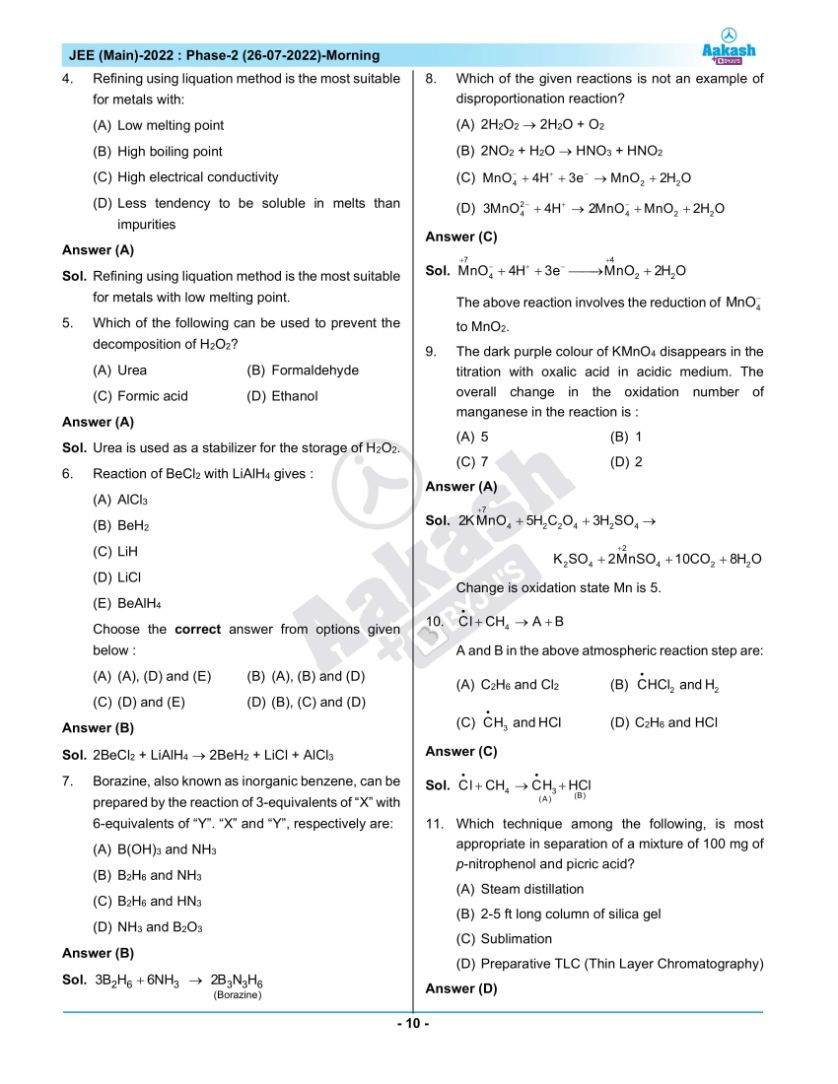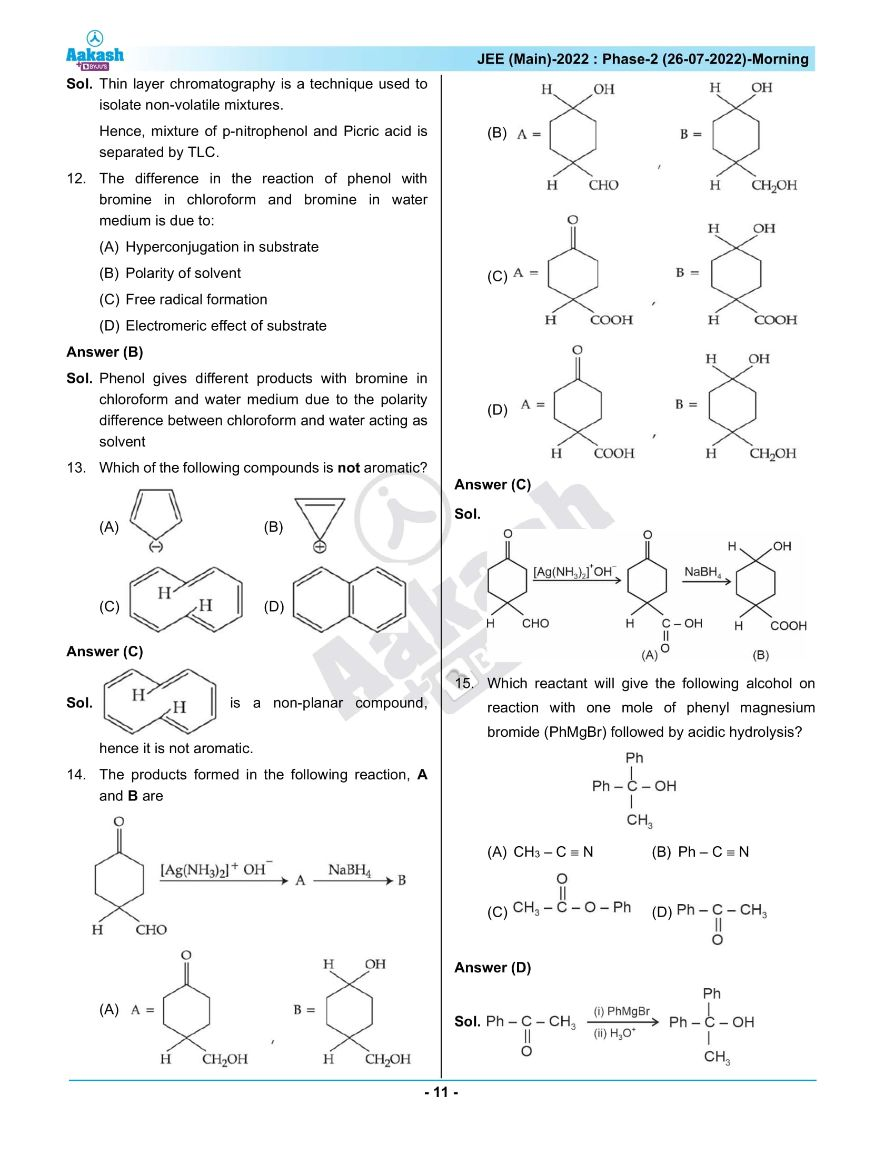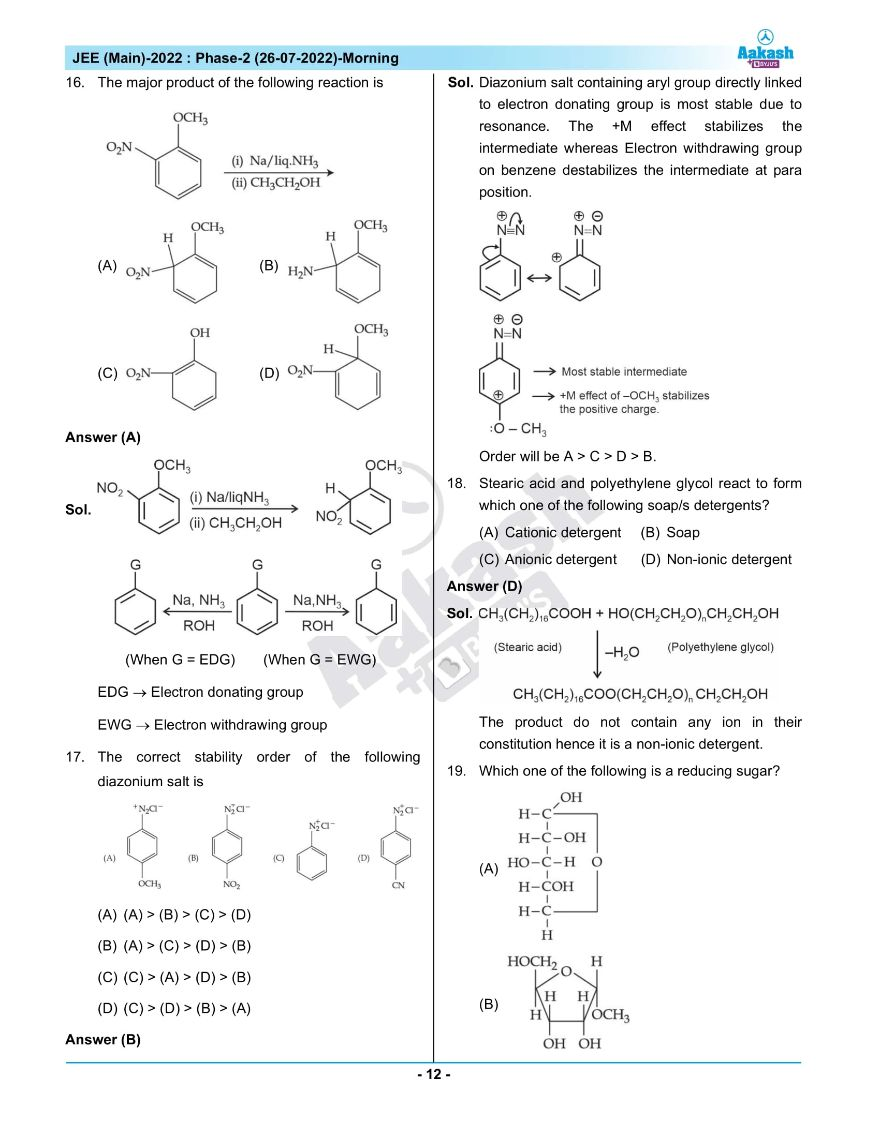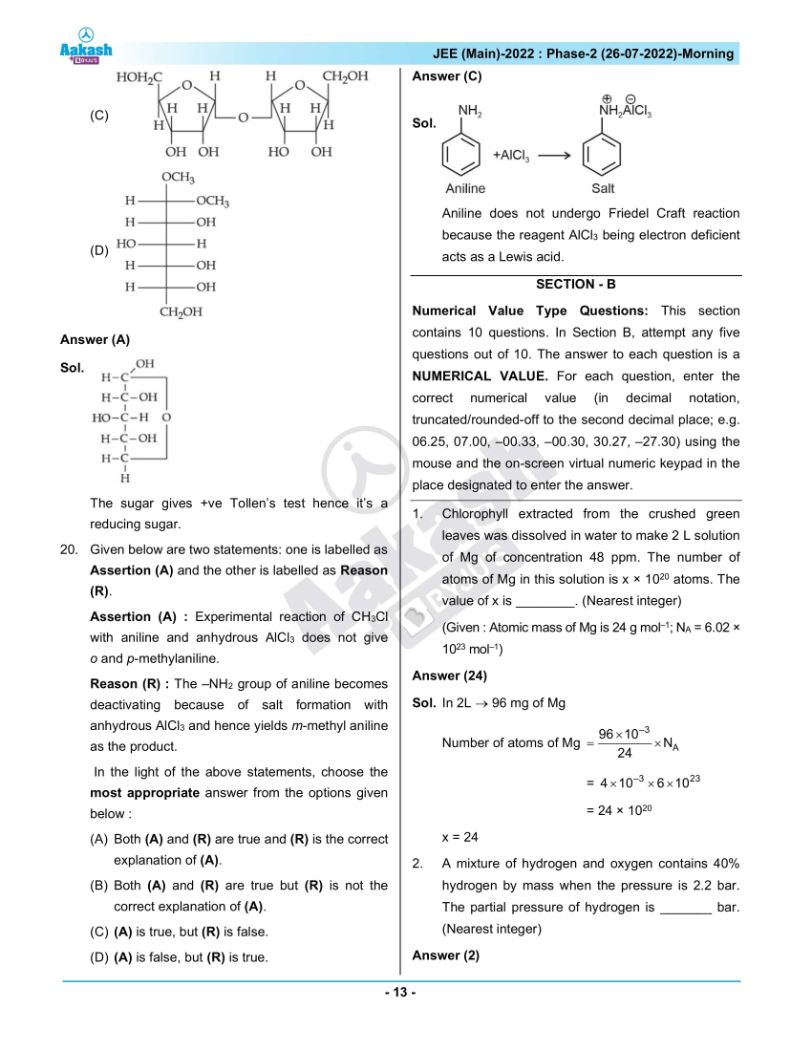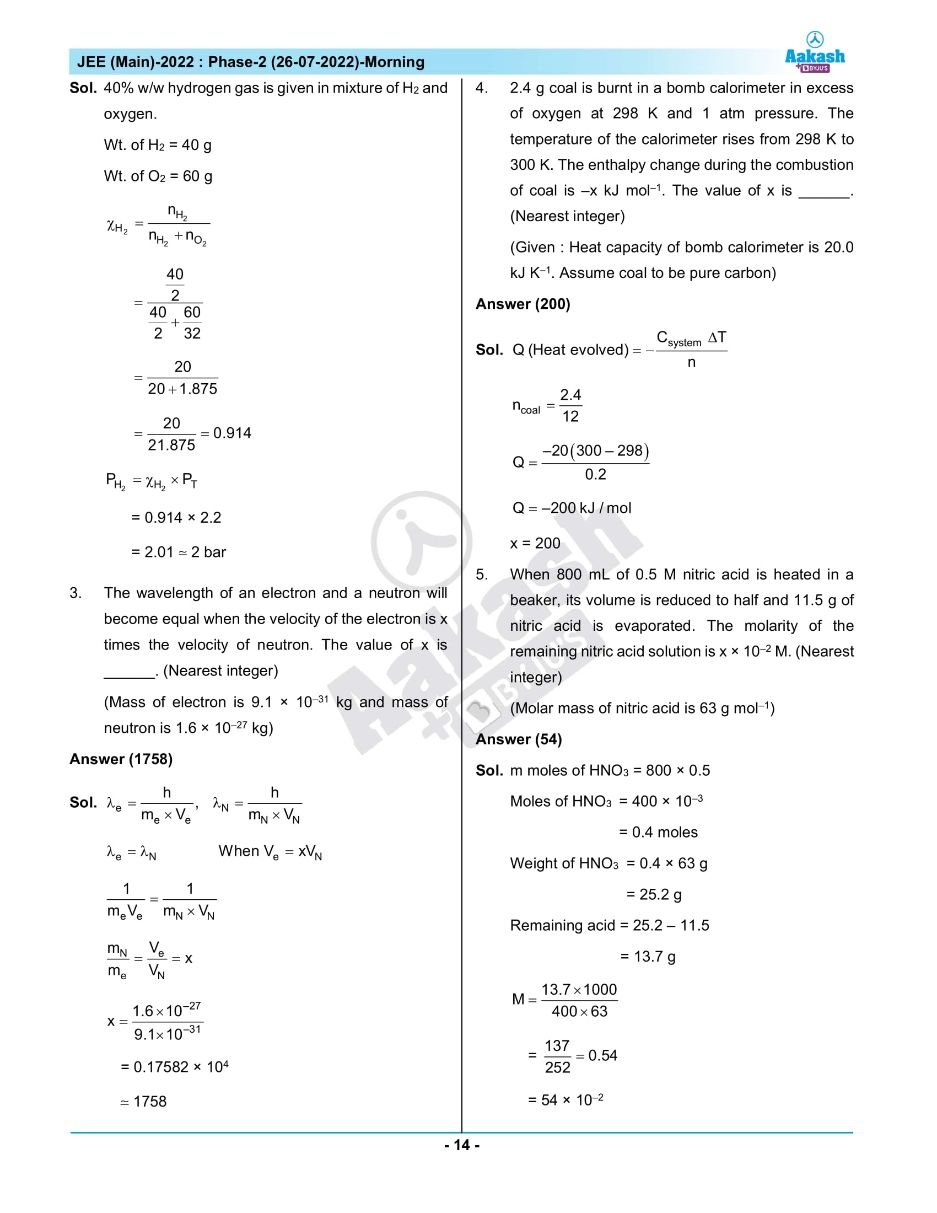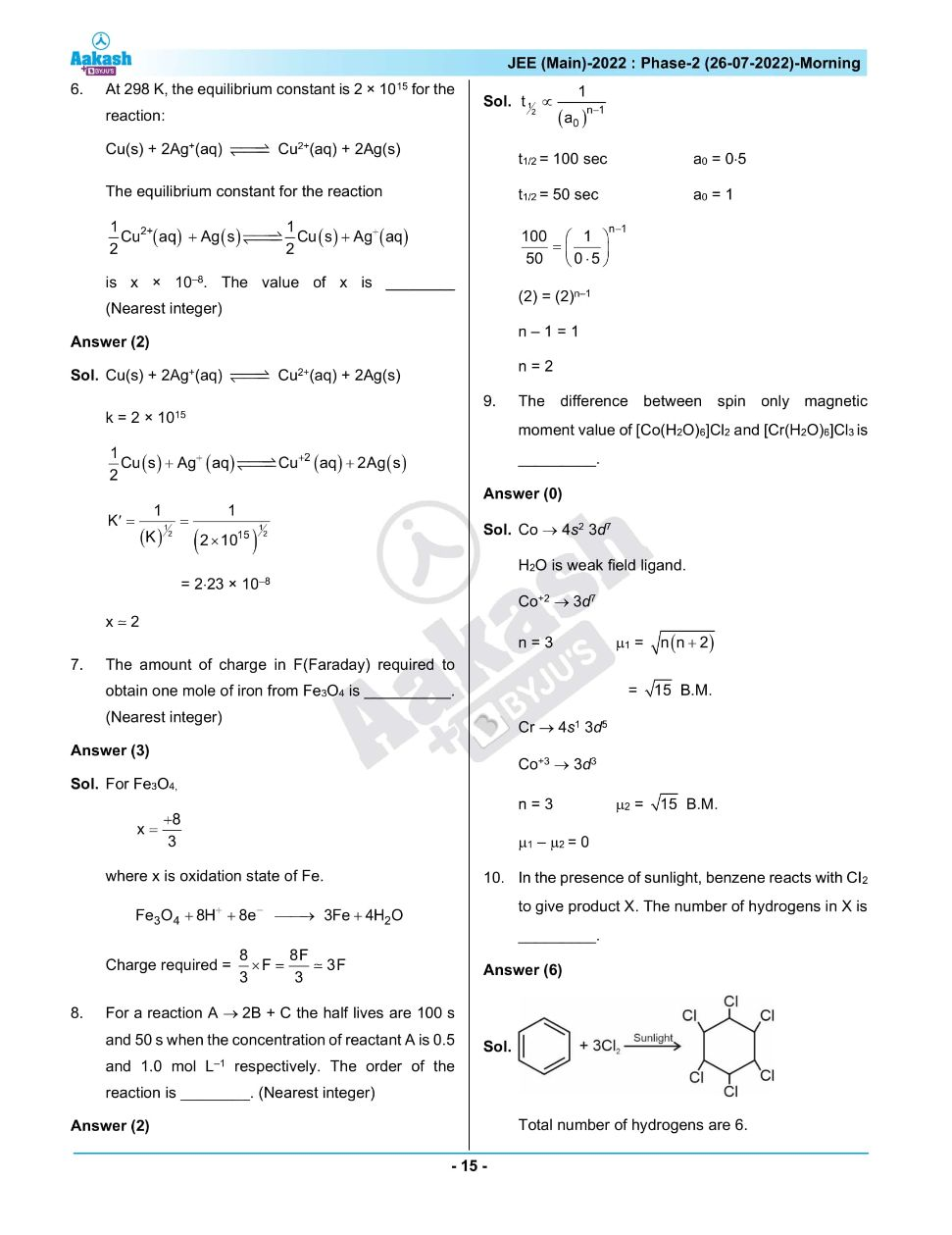## JEE Main 2022 July 26th Shift 1 Paper Analysis